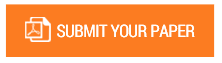Efficient Seed and K Value Selection in K-Means Clustering Using Relative Weight and New Distance Metric

Authors : A.K. Gupta, Premsagar Dandge

Volume/Issue : Volume 2 - 2017, Issue 6 - June

Scribd : https://goo.gl/4C4UkN

Thomson Reuters ResearcherID : https://goo.gl/3bkzwv

K-mean clustering algorithm is used for clustering the data points which are similar to each other. K-means algorithm is popular due to its simplicity and convergence tendency. The general distance metrics used in this algorithm are Euclidean distance, Manhattan distance etc. which are best suited for numeric data like geometric coordinates. These distance metrics does not give full proof result for categorical data. We will be using a new distance metric for calculating the similarity between the categorical data points. The new distance metric uses dynamic attribute weight and frequency probability to differentiate the data points. This ensures the use of categorical properties of the attributes while clustering. The k-mean algorithm needs the information about number of clusters present in the data set in advance before proceeding for cluster analysis. We will be using a different technique for finding out the number of clusters which is based on the data density distribution. In k means algorithm, the initial cluster seeds are selected in a random fashion which may lead to more iteration required for convergent solution. In proposed method, seeds are selected by considering the density distribution which ensures the even distribution of initial seeds. This will reduce the overall iterations required for convergent solution.

Keywords : k-means clustering, categorical data, dynamic attribute weight, frequency probability, data density.

#### CALL FOR PAPERS

Paper Submission Last Date
31 - May - 2022